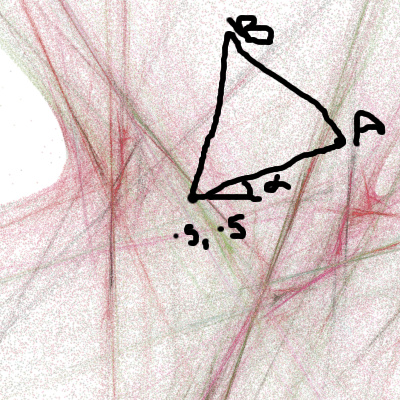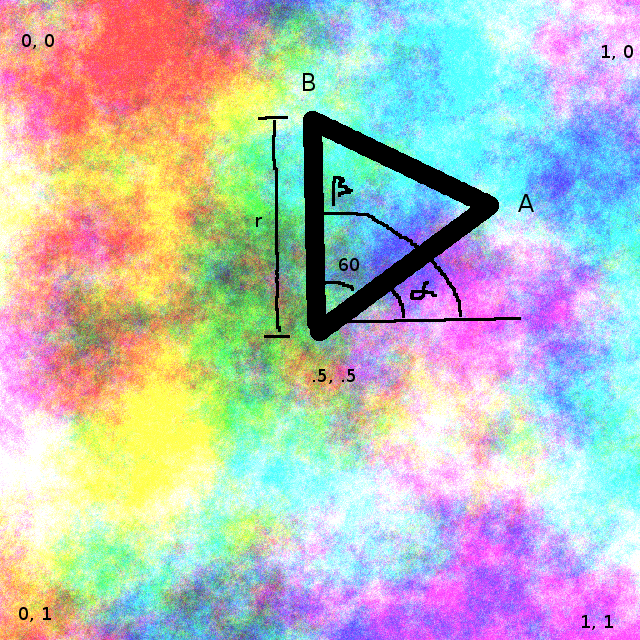#### Howdy, Stranger!

We are about to switch to a new forum software. Until then we have removed the registration on this forum.

# How to use PGraphics for creating textures

edited July 2016

I am trying to make the kaleidscope effect. So far I got it working with a image, but I want to create my own textures. However, I don't get it working. The idea for this approach comes from this site: http://beautifulprogramming.com/endless-kaleidoscope/.

So my question as state in the title, how do you use PGraphics for creating textures? Or is there a better way?

``````Texture texture;

float radius = 300;
float angleStep = 60;

PImage img;

void setup() {
size(800, 600, P3D);
smooth(6);
textureMode(NORMAL);
texture = new Texture();
}

void draw() {
background(255);
texture.update();
hexGrid();
}

void hexGrid() {
noFill();
noStroke();
//stroke(0);

beginShape(TRIANGLE_FAN);
translate(width/2, height/2);
texture(texture.tex);
vertex(0, 0, 0.5, 1); // center
for (int i=0; i<6+1; i++) {
float x = cos(radians(angleStep) * i) * radius;
float y = sin(radians(angleStep) * i) * radius;

if (i%2 == 0) {
vertex(x, y, 0, 0);
} else {
vertex(x, y, 0, 1);
}
}
endShape();
}

class Texture {
PGraphics pg;
PImage tex;

float rot = 0;
float z = 0;
float max = 1000;

Texture() {
pg = createGraphics(width, height, P3D);
pg.rectMode(CENTER);
pg.noStroke();
pg.colorMode(HSB);
}

void update() {
pg.beginDraw();
createTexture();
pg.endDraw();
tex = pg.get();
}

void createTexture() {
float x = 0;
while (x < width + 40) {
float y = 0;
while (y < height + 40) {
float co = 255 * noise(x/500, y/500, z);
fill(co*2, 50, co*3, co*3);
rotRectangle(x, y, 60, rot + x + y);
y += 40;
}
x += 40;
}
z += 0.01;
rot += 0.1;
}

void rotRectangle(float x, float y, float size, float r) {
translate(x, y);
//rotate(r);
beginShape();
vertex(-size/2, -size/2);
vertex(size/2, -size/2);
vertex(size/2, size/2);
vertex(-size/2, size/2);
endShape();
resetMatrix();
}
}
``````
Tagged:

## Answers

• Maybe shaders? It is a long read but it might be a way to manage textures, even in 3D:

https://processing.org/tutorials/pshader/

I hope this helps,

Kf

• the trick to this is the second pair of parameters to vertex to specify what part of the texture is used to fill the hexagon slices

``````vertex(0, 0, 0.5, 1); // center
...
vertex(x, y, 0, 0);
...
vertex(x, y, 0, 1);
``````

your texture doesn't change, the texture coords do... the centre, the first vertex in the fan, can be .5, .5. the other two should be 60 degrees apart and rotate around that centreso B is .5 + r cos(alpha), .5 + r sin(alpha) and A is .5 + r cos(alpha + PI / 3), .5 + r sin(alpha + PI / 3)

``````vertex(0, 0, 0.5, 1); // center
...
vertex(x, y, .5 + r * cos(alpha + PI / 3), .5 + r * sin(alpha + PI / 3));
...
vertex(x, y, .5 + r * cos(alpha), .5 + r * sin(alpha));
``````

where r is .5 if you want to use the whole texture.

and then increment alpha with every frame...

• (sorry, the alpha on the diagram is in the wrong place. i changed my mind later, which is why B comes before A. angle usually starts at 3 o'clock and goes anticlockwise but here it doesn't really matter which way they rotate or where they start as long as A and B are 60 degrees apart.)

• @kfrajer, I did looked at shaders, but that's not what I need and a bit overwhelming.

@koogs, Thanks for the elaborate explanation, it is much appreciated. Although it didn't solve the problem. When I use the image it the textures moves/rotates (I wanted to know how to do that, so that problem is solved, thanks for that), but when I want to use the texture class I get a blank screen.

Below is the code using the texture class.

``````Texture texture;

float radius = 300;
float alpha = 0;
float r = 0.5;

PImage img;

void setup() {
size(800, 600, P3D);
smooth(6);
textureMode(NORMAL);
texture = new Texture();
img = loadImage("mesma.jpg");
}

void draw() {
background(255);
hexShape();
}

void hexShape() {
noFill();
//noStroke();
stroke(0);

beginShape(TRIANGLE_FAN);
translate(width/2, height/2);
texture(texture.tex);
vertex(0, 0, 0.5, 0.5); // center
for (int i=0; i<6+1; i++) {
float angle_deg = 60 * i + 30; //pointy topped
float x = cos(radians(angle_deg)) * radius;
float y = sin(radians(angle_deg)) * radius;

if (i%2 == 0) {
vertex(x, y, .5 + r * cos(alpha + PI / 3), .5 + r * sin(alpha + PI / 3));
} else {
vertex(x, y, .5 + r * cos(alpha), .5 + r * sin(alpha));
}
}
endShape();
alpha += 0.1;
}

class Texture {
PGraphics pg;
PImage tex;

float rot = 0;
float z = 0;
float max = 1000;

Texture() {
pg = createGraphics(width, height, P3D);
pg.rectMode(CENTER);
pg.noStroke();
pg.colorMode(HSB);
}

void update() {
pg.beginDraw();
createTexture();
pg.endDraw();
tex = pg.get();
}

void createTexture() {
float x = 0;
while (x < width + 40) {
float y = 0;
while (y < height + 40) {
float co = 255 * noise(x/500, y/500, z);
fill(co*2, 50, co*3, co*3);
rotRectangle(x, y, 60, rot + x + y);
y += 40;
}
x += 40;
}
z += 0.01;
rot += 0.1;
}

void rotRectangle(float x, float y, float size, float r) {
translate(x, y);
//rotate(r);
beginShape();
vertex(-size/2, -size/2);
vertex(size/2, -size/2);
vertex(size/2, size/2);
vertex(-size/2, size/2);
endShape();
resetMatrix();
}
}
``````
• i changed draw() to the minimal

``````void draw() {
background(255);
texture.update();
image(texture.tex, 0, 0);
noLoop();
//      hexShape();
}
``````

and it turns out that texture.tex isn't set until you call texture.update() (line 67). which you don't.

btw ctrl-t in the editor will indent things nicely.

• rotRectangle() needs to write to pg as well. `vertex` etc isn't enough, needs to be `pg.vertex`

but that's still not enough. i see hexagon, i don't see a texture.

• i think the texture generation thing isn't generating anything interesting. i just see a white box with two lines.

either replace the texture generation code with something that just loads an image and get the hex tiling working. or replace the hex tiling with a single call to `image(texture.tex, 100, 100)`and concentrate on getting the texture generation working.

• That's strange, I copied the code from another sketch.

• @koogs, a late question but could you explain how you came up with this formula?

`.5 + r * cos(alpha + PI / 3)`

• .5 is the middle of the texture

r is the radius (.5 means use the whole rest of the width of the texture)

alpha + PI / 3 is 60 degrees around from alpha, giving you, along with the centre, an equilateral triangle

• But why do you need to multiply r first? Why not

(.5 + r) * cos(alpha + PI / 3) ?

• better picture:textures are 0,0 at top left, 1, 1 at bottom right

so your centre is .5, .5

x = r cos (angle)

so x for A is .5 (because it starts at the centre) plus r cos(alpha)

and x for B is .5 plus r cos(beta). but beta is alpha plus 60 degrees (or PI / 3 radians)

hence .5 + r cos(alpha + PI / 3)

• Thanks man, you're the best

Sign In or Register to comment.